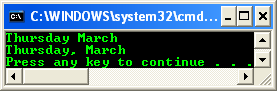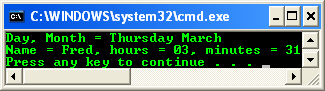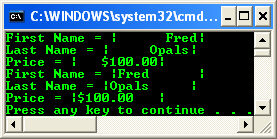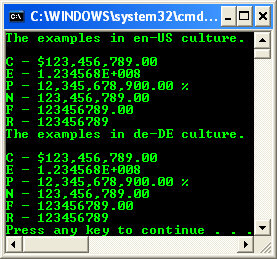# Playing With C++ .NET Formatting 2

The following are the topics available in this part.

2. # Standard Numeric Format Strings

Processing Order

If the value to be formatted is null (Nothing in Visual Basic), an empty string ("") is returned. If the type to be formatted implements the ICustomFormatter interface, the ICustomFormatter::Format method is called. If the preceding step does not format the type, and the type implements the IFormattable interface, the IFormattable::ToString method is called. If the preceding step does not format the type, the type's ToString method, which is inherited from the Object class, is called. Alignment is applied after the preceding steps have been performed. The following code snippet example shows one string created using composite formatting and another created using an object's ToString method. Both types of formatting produce equivalent results.

// testprog.cpp : main project file.

// Format string

#include "stdafx.h"

using namespace System;

using namespace System::Globalization;

int main()

{

String^ FormatString1 = String::Format("{0:dddd MMMM}", DateTime::Now);

Console::WriteLine(FormatString1);

DateTimeFormatInfo^ dfi = gcnew DateTimeFormatInfo();

DateTime dt = DateTime::Now;

dfi->MonthDayPattern = "dddd, MMMM";

Console::WriteLine(dt.ToString("m", dfi));

return 0;

}

Output:Assuming that the current day is a Thursday in May, the value of both strings in the preceding example is Thursday May in the U.S. English culture. Console::WriteLine exposes the same functionality as String::Format. The only difference between the two methods is that String::Format returns its result as a string, while Console::WriteLine writes the result to the output stream associated with the Console object. The following example uses the Console::WriteLine method to format the value of MyInt to a currency value.

int MyInt = 100;

Console::WriteLine("{0:C}", MyInt);

This code displays \$100.00 to the console on computers that have U.S. English as the current culture.

The following example demonstrates formatting multiple objects, including formatting one object two different ways.

// testprog.cpp : main project file.

#include "stdafx.h"

using namespace System;

int main()

{

String^ myName = "Fred";

String^ FormatString1 = String::Format("Day, Month = {0:dddd MMMM}", DateTime::Now);

Console::WriteLine("{0}", FormatString1);

Console::WriteLine("Name = {0}, hours = {1:hh}, minutes = {1:mm}", myName, DateTime::Now);

return 0;

}

The output from the preceding example is:The following example demonstrates the use of alignment in formatting. The arguments that are formatted are placed between vertical bar characters (|) to highlight the resulting alignment.

// testprog.cpp : main project file.

#include "stdafx.h"

using namespace System;

int main()

{

String^ myFName = "Fred";

String^ myLName = "Opals";

int myInt = 100;

String^ FormatFName = String::Format("First Name = |{0,10}|", myFName);

String^ FormatLName = String::Format("Last Name = |{0,10}|", myLName);

String^ FormatPrice = String::Format("Price = |{0,10:C}|", myInt);

Console::WriteLine("{0}", FormatFName);

Console::WriteLine("{0}",FormatLName);

Console::WriteLine("{0}",FormatPrice);

FormatFName = String::Format("First Name = |{0,-10}|", myFName);

FormatLName = String::Format("Last Name = |{0,-10}|", myLName);

FormatPrice = String::Format("Price = |{0,-10:C}|", myInt);

Console::WriteLine("{0}",FormatFName);

Console::WriteLine("{0}",FormatLName);

Console::WriteLine("{0}",FormatPrice);

return 0;

}

The preceding code displays the following to the console in the U.S. English culture. Different cultures display different currency symbols and separators.Standard Numeric Format Strings

Standard numeric format strings are used to format common numeric types. A standard format string takes the form Axx where A is a single alphabetic character called the format specifier, and xx is an optional integer called the precision specifier. The format specifier must be one of the built-in format characters. The precision specifier ranges from 0 to 99 and controls the number of significant digits or zeros to the right of a decimal. The format string cannot contain white spaces. If the format string does not contain one of the standard format specifiers, then a FormatException is thrown. For example, the format string "z" is interpreted as a standard numeric format string because it contains one alphabetic character, but the alphabetic character is not one of the standard numeric format specifiers so a FormatException is thrown. Any numeric format string that does not fit the definition of a standard numeric format string is interpreted as a custom numeric format string. The format string "c!" is interpreted as a custom format string because it contains two alphabetic characters, even though the character "c" is a standard numeric format specifier. The following table describes the standard numeric format strings. Note that the result string produced by these format specifiers is influenced by the settings in the Regional Options control panel. Computers using different settings will generate different result strings.

 Format specifier Name Description C or c Currency The number is converted to a string that represents a currency amount. The conversion is controlled by the currency format information of the NumberFormatInfo object used to format the number. The precision specifier indicates the desired number of decimal places. If the precision specifier is omitted, the default currency precision given by the NumberFormatInfo is used. D or d Decimal This format is supported for integral types only. The number is converted to a string of decimal digits (0-9), prefixed by a minus sign if the number is negative. The precision specifier indicates the minimum number of digits desired in the resulting string. If required, the number is padded with zeros to its left to produce the number of digits given by the precision specifier. E or e Scientific (exponential) The number is converted to a string of the form "-d.ddd…E+ddd" or "-d.ddd...e+ddd", where each 'd' indicates a digit (0-9). The string starts with a minus sign if the number is negative. One digit always precedes the decimal point. The precision specifier indicates the desired number of digits after the decimal point. If the precision specifier is omitted, a default of six digits after the decimal point is used. The case of the format specifier indicates whether to prefix the exponent with an 'E' or an 'e'. The exponent always consists of a plus or minus sign and a minimum of three digits. The exponent is padded with zeros to meet this minimum, if required. F or f Fixed-point The number is converted to a string of the form "-ddd.ddd…" where each 'd' indicates a digit (0-9). The string starts with a minus sign if the number is negative. The precision specifier indicates the desired number of decimal places. If the precision specifier is omitted, the default numeric precision given by the NumberFormatInfo is used. G or g General The number is converted to the most compact of either fixed-point or scientific notation, depending on the type of the number and whether a precision specifier is present. If the precision specifier is omitted or zero, the type of the number determines the default precision, as indicated by the following list.   Byte or SByte: 3 Int16 or UInt16: 5 Int32 or UInt32: 10 Int64 or UInt64: 19 Single: 7 Double: 15 Decimal: 29   Fixed-point notation is used if the exponent that would result from expressing the number in scientific notation is greater than -5 and less than the precision specifier; otherwise, scientific notation is used. The result contains a decimal point if required and trailing zeroes are omitted. If the precision specifier is present and the number of significant digits in the result exceeds the specified precision, then the excess trailing digits are removed by rounding. If scientific notation is used, the exponent in the result is prefixed with 'E' if the format specifier is 'G', or 'e' if the format specifier is 'g'. The exception to the preceding rule is if the number is a Decimal and the precision specifier is omitted. In that case, fixed-point notation is always used and trailing zeroes are preserved. N or n Number The number is converted to a string of the form "-d,ddd,ddd.ddd...", where each 'd' indicates a digit (0-9). The string starts with a minus sign if the number is negative. Thousand separators are inserted between each group of three digits to the left of the decimal point. The precision specifier indicates the desired number of decimal places. If the precision specifier is omitted, the default numeric precision given by the NumberFormatInfo is used. P or p Percent The number is converted to a string that represents a percent as defined by the NumberFormatInfo.PercentNegativePattern property or the NumberFormatInfo.PercentPositivePattern property. If the number is negative, the string produced is defined by the PercentNegativePattern and starts with a minus sign. The converted number is multiplied by 100 in order to be presented as a percentage. The precision specifier indicates the desired number of decimal places. If the precision specifier is omitted, the default numeric precision given by NumberFormatInfo is used. R or r Round-trip The round-trip specifier guarantees that a numeric value converted to a string will be parsed back into the same numeric value. When a numeric value is formatted using this specifier, it is first tested using the general format, with 15 spaces of precision for a Double and 7 spaces of precision for a Single. If the value is successfully parsed back to the same numeric value, it is formatted using the general format specifier. However, if the value is not successfully parsed back to the same numeric value, then the value is formatted using 17 digits of precision for a Double and 9 digits of precision for a Single. Although a precision specifier can be appended to the round-trip format specifier, it is ignored. Round trips are given precedence over precision when using this specifier. This format is supported by floating-point types only. X or x Hexadecimal The number is converted to a string of hexadecimal digits. The case of the format specifier indicates whether to use uppercase or lowercase characters for the hexadecimal digits greater than 9. For example, use 'X' to produce "ABCDEF", and 'x' to produce "abcdef". The precision specifier indicates the minimum number of digits desired in the resulting string. If required, the number is padded with zeros to its left to produce the number of digits given by the precision specifier. This format is supported for integral types only. Table 1

Try the following example. Note that the regional and language options of the machine that runs this example must be set to a suitable culture to correctly display of the euro sign

// testprog.cpp : main project file.

#include "stdafx.h"

using namespace System;

using namespace System::Globalization;

int main()

{

CultureInfo^ CurrentCulture;

CurrentCulture = gcnew CultureInfo("en-us");

double MyDouble = 123456789;

Console::WriteLine("The examples in en-US culture.\n");

Console::WriteLine("C - {0}", MyDouble.ToString("C"));

Console::WriteLine("E - {0}", MyDouble.ToString("E"));

Console::WriteLine("P - {0}", MyDouble.ToString("P"));

Console::WriteLine("N - {0}", MyDouble.ToString("N"));

Console::WriteLine("F - {0}", MyDouble.ToString("F"));

Console::WriteLine("R - {0}", MyDouble.ToString("R"));

CurrentCulture = gcnew CultureInfo("de-DE");

Console::WriteLine("The examples in de-DE culture.\n");

Console::WriteLine("C - {0}", MyDouble.ToString("C"));

Console::WriteLine("E - {0}", MyDouble.ToString("E"));

Console::WriteLine("P - {0}", MyDouble.ToString("P"));

Console::WriteLine("N - {0}", MyDouble.ToString("N"));

Console::WriteLine("F - {0}", MyDouble.ToString("F"));

Console::WriteLine("R - {0}", MyDouble.ToString("R"));

return 0;

}

Output: